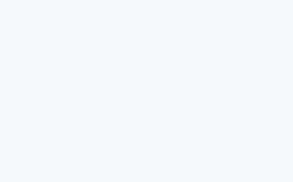# python库介绍-图像处理工具pillow中文文档-手册(2018 5.*)

## 概述

Python Imaging Library给Python解释器增加了图像处理能力。

• 图像存储

PIL适合图像归档和图像批量处理，你可以使用它建立缩略图，转换格式，打印图片等。

• 图像显示

• 图像处理

## 使用 Image 类

PIL最重要的类是Image, 你可以通过多种方法创建这个类的实例，比如从文件加载图像，或者处理其他图像, 或者从或从头开始创建图像。

``>>> from PIL import Image>>> im = Image.open("hopper.ppm")``

``>>> print(im.format, im.size, im.mode)PPM (512, 512) RGB``

format属为图像来源。如果不是从文件读取就是None。size属性是包含宽度和高度(像素)的二元组）。模式属性定义了图像中波段的数量和名称，以及像素类型和深度。 常见模式为灰度图像为“L”（亮度），真彩色图像为“RGB”，印刷四色为“CMYK”。

``>>> im.show()``

show的标准版本效率不高，因为它将图像保存到临时文件并调用xv工具显示图像。 如果你没有安装xv，它甚至不会工作。 它对于调试和测试非常方便。

``from PIL import Imageim = Image.open("demo.jpg")print(im.format, im.size, im.mode)im.show()``image.png

## 读写图像

PIL库支持大量图片格式。使用Image模块的open()函数从磁盘读取文件。你不需要知道文件格式就能打开它，PIL能够根据文件内容自动确定确定文件格式。

``import os, sysfrom PIL import Imagefor infile in sys.argv[1:]:    f, e = os.path.splitext(infile)    outfile = f + ".jpg"    if infile != outfile:        try:            Image.open(infile).save(outfile)        except IOError:            print("cannot convert", infile)``

save()方法的第二个参数可以指定文件格式，如果你使用非标准的扩展名你必须这样做。

``import os, sysfrom PIL import Imagesize = (128, 128)for infile in sys.argv[1:]:    outfile = os.path.splitext(infile) + ".thumbnail"    if infile != outfile:        try:            im = Image.open(infile)            im.thumbnail(size)            im.save(outfile, "JPEG")        except IOError:            print("cannot create thumbnail for", infile)``

``import sysfrom PIL import Imagefor infile in sys.argv[1:]:    try:        with Image.open(infile) as im:            print(infile, im.format, "%dx%d" % im.size, im.mode)    except IOError:        pass``

## 剪切，粘贴，合并图像

Image类包含允许您操作图像内区域的方法。要从图像中提取子矩形，请使用crop()方法。

``box = (100, 100, 400, 400)region = im.crop(box)``

``region = region.transpose(Image.ROTATE_180)im.paste(region, box)``

``def roll(image, delta):    """Roll an image sideways."""    xsize, ysize = image.size    delta = delta % xsize    if delta == 0: return image    part1 = image.crop((0, 0, delta, ysize))    part2 = image.crop((delta, 0, xsize, ysize))    part1.load()    part2.load()    image.paste(part2, (0, 0, xsize-delta, ysize))    image.paste(part1, (xsize-delta, 0, xsize, ysize))    return image``

pillow_roll.py

``from PIL import Imagedef roll(image, delta):    """Roll an image sideways."""    xsize, ysize = image.size    delta = delta % xsize    if delta == 0: return image    part1 = image.crop((0, 0, delta, ysize))    part2 = image.crop((delta, 0, xsize, ysize))    part1.load()    part2.load()    image.paste(part2, (0, 0, xsize-delta, ysize))    image.paste(part1, (xsize-delta, 0, xsize, ysize))    return imageim = Image.open("demo.jpg")im = roll(im,100)im.show()``image.png

PIL还允许您使用多波段图像的各个波段，例如RGB图像。拆分方法会创建新图像，每个图像都包含原始多波段图像中的一个波段。合并功能采用模式和图像元组，并将它们组合成新图像。以下示例交换RGB图像的三个波段：

``r, g, b = im.split()im = Image.merge("RGB", (b, g, r))``

## 几何变换

PIL.Image.Image类包含调整resize()和rotate()图像的方法。前者需要给出尺寸的元组，后者是逆时针方向的角度。

``out = im.resize((128, 128))out = im.rotate(45) # degrees counter-clockwise``

``out = im.transpose(Image.FLIP_LEFT_RIGHT)out = im.transpose(Image.FLIP_TOP_BOTTOM)out = im.transpose(Image.ROTATE_90)out = im.transpose(Image.ROTATE_180)out = im.transpose(Image.ROTATE_270)``

``#!/usr/bin/python3# -*- coding: utf-8 -*-# Author:    xurongzhong#126.com 技术支持qq群：6089740# CreateDate: 2018-3-27# rotate.pyimport globimport os from PIL import Imagedef rotate(files, dst, value=90):    for file_ in files:        img = Image.open(file_)        img = img.rotate(value)        name = "{}{}{}".format(dst, os.sep, os.path.basename(file_))        img.save(name)src = r'/home/andrew/code/tmp_photos'dst = r'/home/andrew/code/tmp_photos2'common = glob.glob('{}{}*.*'.format(src, os.sep))  rotate(common, dst)``

## 颜色转换

PIL允许您使用convert() 方法在不同的像素表示之间转换图像。

## 模式转换

``from PIL import Imageim = Image.open("hopper.ppm").convert("L")``

## 图像增强

PIL提供了许多可用于增强图像的方法和模块。

• 过滤器

ImageFilter模块包含许多可以与filter()方法一起使用的预定义增强过滤器。

``from PIL import ImageFilterout = im.filter(ImageFilter.DETAIL)``
• 点操作
point()方法可用于转换图像的像素值(例如图像对比度操作)。在大多数情况下，期望一个参数的函数对象可以传递给此方法。每个像素都根据该功能进行处理：
``# multiply each pixel by 1.2out = im.point(lambda i: i * 1.2)``

``# split the image into individual bandssource = im.split()R, G, B = 0, 1, 2# select regions where red is less than 100mask = source[R].point(lambda i: i < 100 and 255)# process the green bandout = source[G].point(lambda i: i * 0.7)# paste the processed band back, but only where red was < 100source[G].paste(out, None, mask)# build a new multiband imageim = Image.merge(im.mode, source)``

Python仅根据确定结果所需的逻辑表达式部分进行评估，并返回检查的最后值作为表达式的结果。因此，如果上面的表达式为假（0），Python不会查看第二个操作数，并返回0.否则，返回255。

• 增强

``from PIL import ImageEnhanceenh = ImageEnhance.Contrast(im)enh.enhance(1.3).show("30% more contrast")``

## 图像序列

Python图像库包含对图像序列（也称为动画格式）的一些基本支持。支持的序列格式包括FLI/FLC, GIF和一些实验格式。 TIFF文件也可以包含多个帧。

``from PIL import Imageim = Image.open("animation.gif")im.seek(1) # skip to the second frametry:    while 1:        im.seek(im.tell()+1)        # do something to imexcept EOFError:    pass # end of sequence``

``from PIL import ImageSequencefor frame in ImageSequence.Iterator(im):    # ...do something to frame...``

Python影像库包括在Postscript打印机上打印图像，文本和图形的功能。这里有一个简单的例子：

``from PIL import Imagefrom PIL import PSDrawim = Image.open("hopper.ppm")title = "hopper"box = (1*72, 2*72, 7*72, 10*72) # in pointsps = PSDraw.PSDraw() # default is sys.stdoutps.begin_document(title)# draw the image (75 dpi)ps.image(box, im, 75)ps.rectangle(box)# draw titleps.setfont("HelveticaNarrow-Bold", 36)ps.text((3*72, 4*72), title)ps.end_document()``

``from PIL import Imageim = Image.open("hopper.ppm")``

``from PIL import Imagewith open("hopper.ppm", "rb") as fp:    im = Image.open(fp)``

``import StringIOim = Image.open(StringIO.StringIO(buffer))``

``from PIL import Image, TarIOfp = TarIO.TarIO("Tests/images/hopper.tar", "hopper.jpg")im = Image.open(fp)``

## 控制解码器

draft() 方法处理已打开但尚未加载的图像，以便尽可能匹配给定的模式和大小。这是通过重新配置图像解码器完成的。

``from PIL import Imagefrom __future__ import print_functionim = Image.open(file)print("original =", im.mode, im.size)im.draft("L", (100, 100))print("draft =", im.mode, im.size)``

``original = RGB (512, 512)draft = L (128, 128)``

## 教你几招，让你vim编辑器变得友善起来(特别水，不要点进来)

2018-9-10 9:43:00

## 还在用百度？来试试这10个良心的搜索引擎吧

2018-9-10 12:00:00

0 条回复 A文章作者 M管理员
暂无讨论，说说你的看法吧